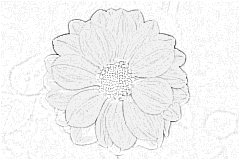# DoGFilter

This filter finds edges using the Difference of Gaussians method. This simply means blurring the image twice with a different radius each time and then subtracting one blurred image from the other. It produces a variety of effects depending on the two radii given. There are option to invert or normalize the output, useful when the edges ar every faint.DoGFilter